Ex 9.3

Chapter 9 Class 11 Sequences and Series (Term 1)
Serial order wise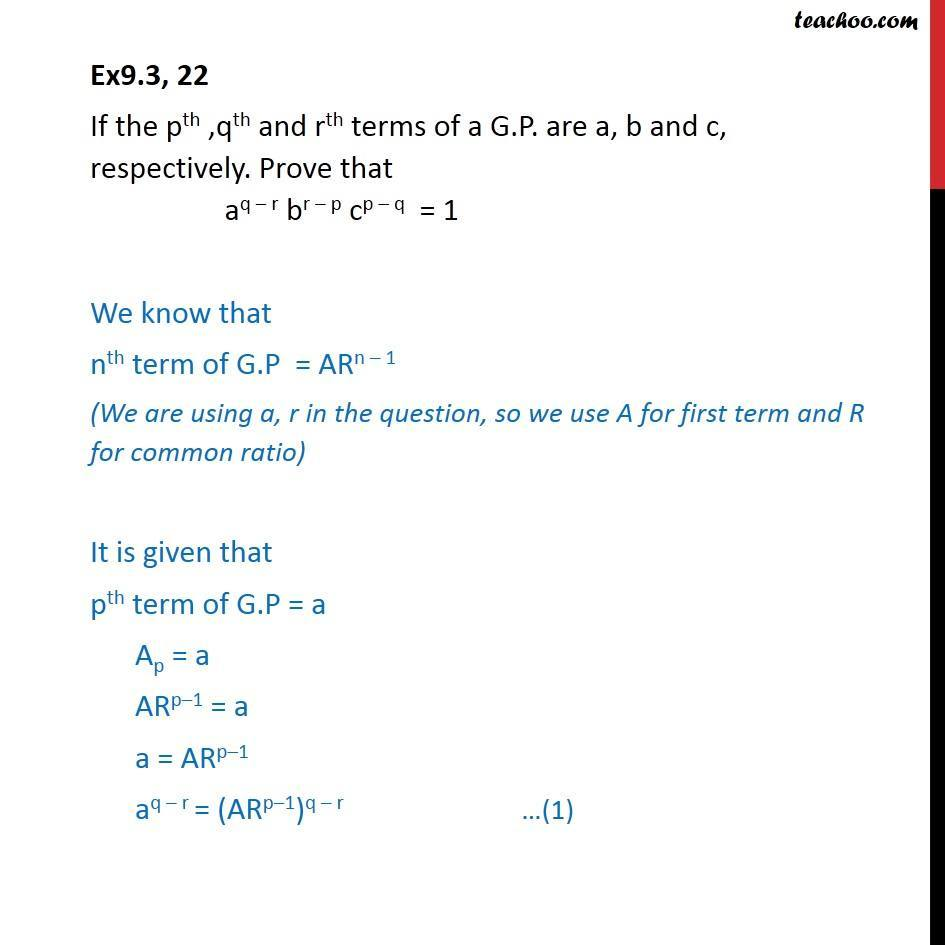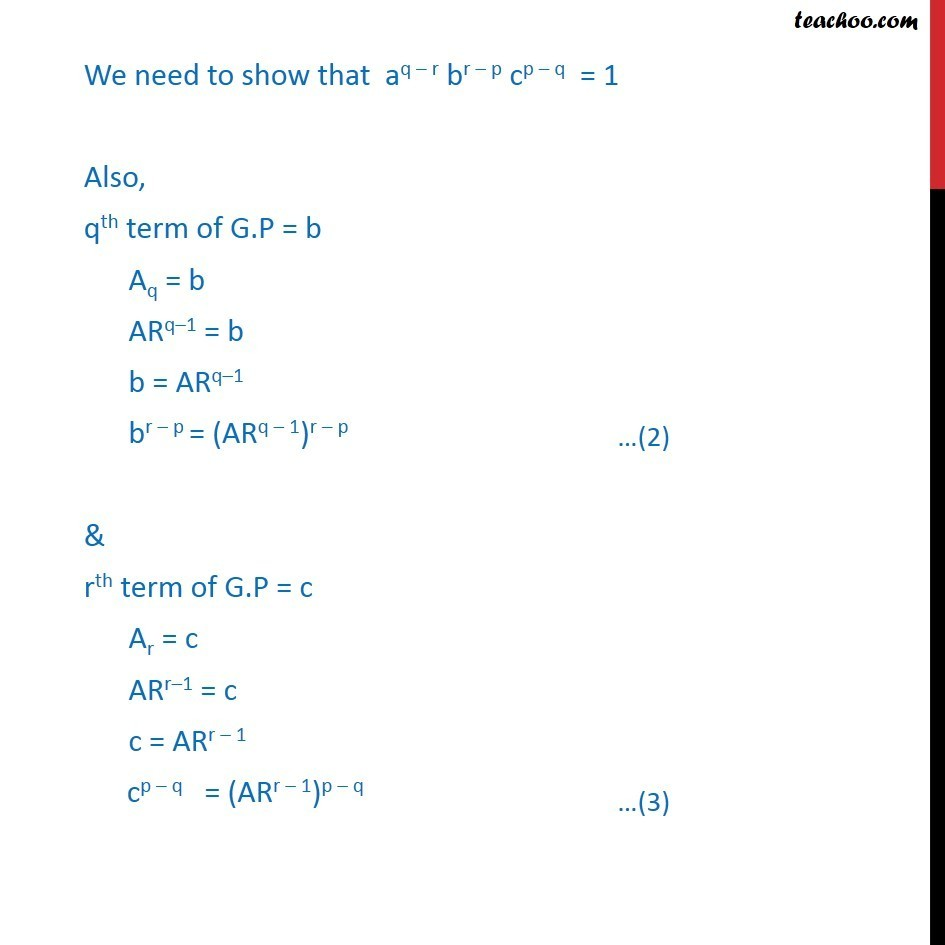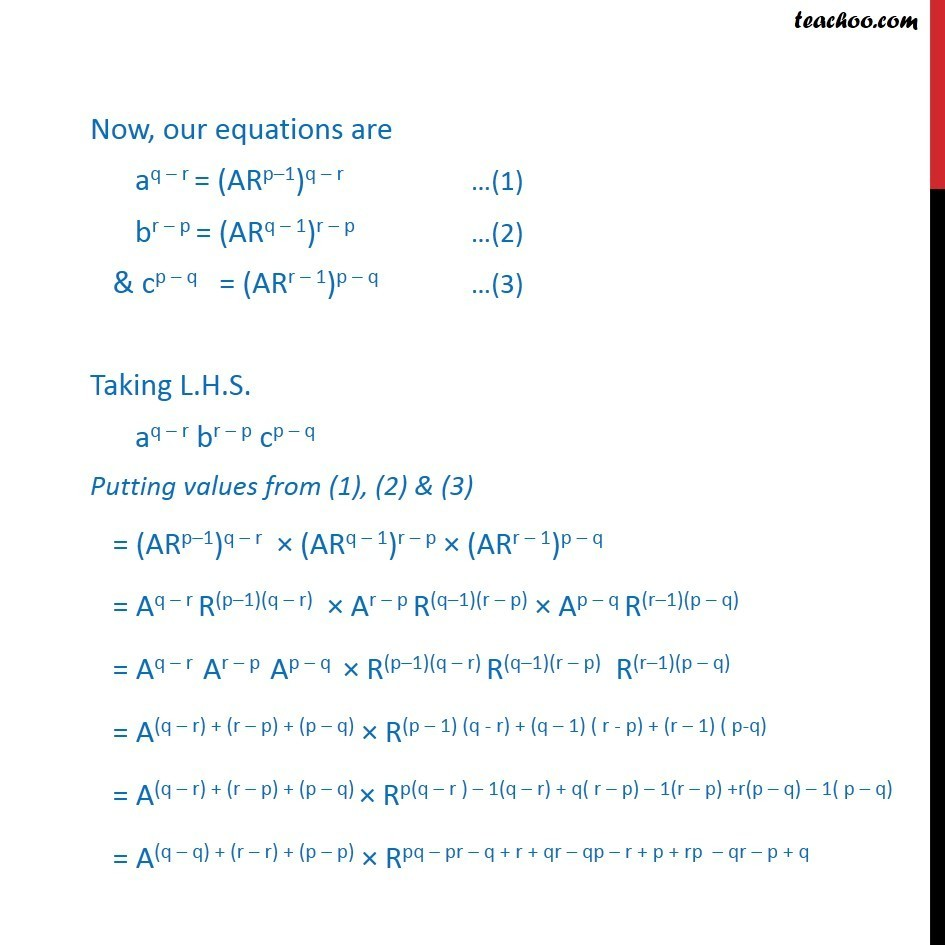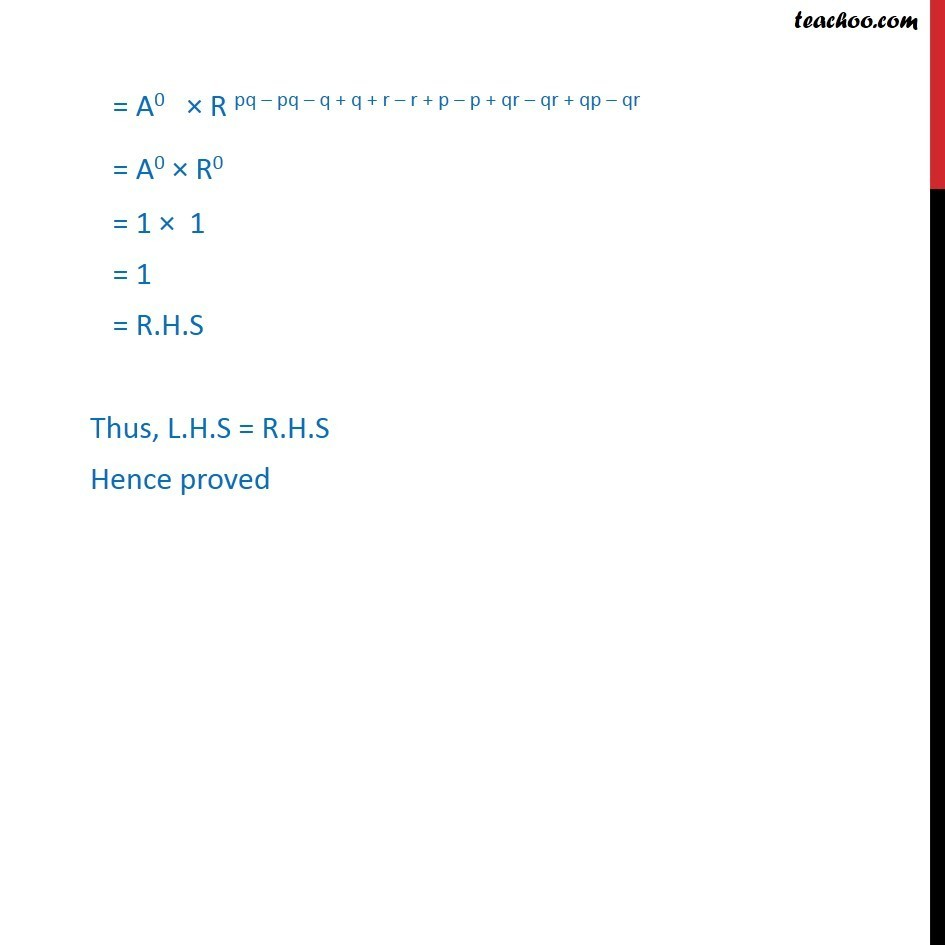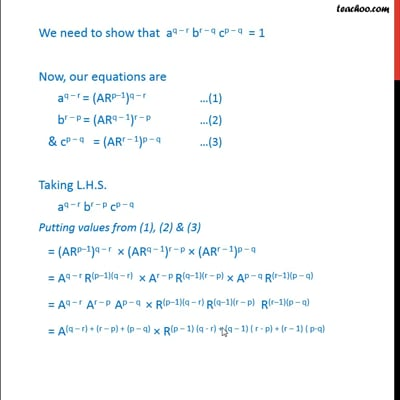This video is only available for Teachoo black users

### Transcript

Ex9.3, 22 If the pth ,qth and rth terms of a G.P. are a, b and c, respectively. Prove that aq r br p cp q = 1 We know that nth term of G.P = ARn 1 (We are using a, r in the question, so we use A for first term and R for common ratio) It is given that pth term of G.P = a Ap = a ARp 1 = a a = ARp 1 aq r = ("ARp 1")q r We need to show that aq r br p cp q = 1 Also, qth term of G.P = b Aq = b ARq 1 = b b = ARq 1 br p = (ARq 1)r p & rth term of G.P = c Ar = c ARr 1 = c c = ARr 1 cp q = (ARr 1)p q Now, our equations are aq r = ("ARp 1")q r (1) br p = (ARq 1)r p (2) & cp q = (ARr 1)p q (3) Taking L.H.S. aq r br p cp q Putting values from (1), (2) & (3) = ("ARp 1")q r (ARq 1)r p (ARr 1)p q = "Aq r R(p 1")(q r) "Ar p R(q 1")(r p) "Ap q R(r 1")(p q) = "Aq r Ar p Ap q " "R(p 1")(q r) "R(q 1")(r p) "R(r 1")(p q) = A(q r) + (r p) + (p q) R(p 1) (q - r) + (q 1) ( r - p) + (r 1) ( p-q) = A(q r) + (r p) + (p q) Rp(q r ) 1(q r) + q( r p) 1(r p) +r(p q) 1( p q) = A(q q) + (r r) + (p p) Rpq pr q + r + qr qp r + p + rp qr p + q = A0 R pq pq q + q + r r + p p + qr qr + qp qr = A0 R0 = 1 1 = 1 = R.H.S Thus, L.H.S = R.H.S Hence proved